# Test: Arun Sharma Based Level 3: Time & Work

## 30 Questions MCQ Test Quantitative Aptitude for Banking Preparation | Test: Arun Sharma Based Level 3: Time & Work

Description
Attempt Test: Arun Sharma Based Level 3: Time & Work | 30 questions in 25 minutes | Mock test for Banking Exams preparation | Free important questions MCQ to study Quantitative Aptitude for Banking Preparation for Banking Exams Exam | Download free PDF with solutions
QUESTION: 1

### A can lay railway track between two given stations in 16 days and B can do the same job in 12 days. With help of C, they did the job in 4 days only. Then, C alone can do the job in:

Solution:

(A + B + C)'s 1 day's work = 1/4
A's 1 day's work = 1/16
B's 1 day's work = 1/12
∴ C's 1 day's work = 1/4 - (1/16 + 1/12) = 1/4 - 7/48 = 5/48
So, C alone can do the work in 48/5 days

QUESTION: 2

### A and B can do a piece of work in 30 days, while B and C can do the same work in 24 days and C and A in 20 days. They all work together for 10 days when B and C leave. How many days more will A take to finish the work?

Solution:

2(A + B + C)'s 1 clay's work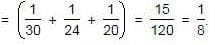Therefore. (A + B + C)'s 1 clay's work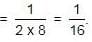Work done by A, B. C in 10 days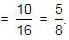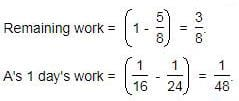Now, 1/48 work is done by A in 1 day.
So. 3/8 work will be done by A in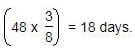QUESTION: 3

### Ravi and Kumar are working on an assignment. Ravi takes 6 hours to type 32 pages on a computer, while Kumar takes 5 hours to type 40 pages. How much time will they take, working together on two different computers to type an assignment of 110 pages?

Solution:

► Number of pages typed by Ravi in 1 hour = 32/6 = 16/3
► Number of pages typed by kumar in 1 hour = 40/5 = 8
► Number of pages typed by both in 1 hour = 16/3 + 8 = 40/3
∴ Time taken by both to type 110 pages = 110 x 3/40 hours
⇒ 33/4 hours or 8 hours 15 minutes

QUESTION: 4

A alone can do a piece of work in 6 days and B alone in 8 days. A and B undertook to do it for Rs. 3200. With the help of C, they completed the work in 3 days. How much is to be paid to C?

Solution:

C's 1 day's work = 1/3 - (1/6 + 1/8)
⇒ 1/3 - 7/24
⇒ 1/24
A's wages : B's wages : C's wages = 1/6 : 1/8 : 1/24
⇒ 4 : 3 : 1
C's share (for 3 days) = Rs.(3 * 1/24 * 3200)
⇒ Rs. 400

QUESTION: 5

X and Y can do a piece of work in 20 days and 12 days respectively. X started the work alone and then after 4 days Y joined him till the completion of the work. How long did the work last?

Solution:

Work done by X in 4 days = (1/20) * 4 = 1/5
Remaining work =1 - 1/5 = 4/5
(X + Y)'s 1 day's work = 1/20 + 1/12 = 8/60 = 2/15
Now, 2/15 work is done by X and Y in 1 day.
So, 4/5 work will be done by X and Y in (15/2) * (4/5) = 6 days.
Hence, total time taken = (6 + 4) days = 10 days.

QUESTION: 6

A can do a work in 15 days and B in 20 days. If they work on it together for 4 days, then the fraction of the work that is left is :

Solution:

► A can do work in 15 days therefore amount of work done by A in 1 day is 60/15 = 4 units
► B can do work in 15 days therefore amount of work done by A in 1 day is 60/20 = 3 units
► Total amount of work done in 1 day = A work of 1 day + B's work of 1 day = 4 units + 3 units = 7 units
► Amount of work done in 4 days = 4*7 = 28 units
Amount of Work Left = 60 - 28 = 32 units
► Fraction of work left = Amount of work left/ Total Work = 32/60
⇒ 8/15.

QUESTION: 7

A works twice as fast as B. If B can complete a work in 12 days independently, the number of days in which A and B can together finish the work in

Solution:

Ratio of rates of working of A and B = 2 : 1
So, ratio of time taken = 1 : 2
B's 1 day's work = 1/12
∴ A's one day work = 1/3 (2 times of B's work)
(A + B)'s 1 day's work = (1/6) + (1/12) = 3/12 = 1/4
So, A and B together can finish work in 4 days.

QUESTION: 8

If A and B together can complete a piece of work in 15 days and B alone in 20 days, in how many days can A alone complete the work?

Solution:

Given, (A + B)’s 1 day’s work = 1/15
B’s 1 day’s work  = 1/20
∴ A’s 1 day’s work will be
⇒ 1/15 - 1/20
⇒ (4-3)/60
⇒ 1/60
∴ A alone will do the work in 60 days.

QUESTION: 9

A can do a piece of work in 4 hours; B and C together can do it in 3 hours, while A and C together can do it in 2 hours. How long will B alone take to do it?

Solution:

A’s 1 hour work = 1/4
(B + C)’s 1 hour work = 1/3
(C + A)’s 1 hour work = 1/2
(A + B + C)’s 1 hour work = 1/4 + 1/3 =7/12
B’s 1 hour work = 7/12 - 1/2 = 1/12
B alone can do a piece of work in 12 hours.

QUESTION: 10

A can finish a work in 18 days and B can do the same work in 15 days. B worked for 10 days and left the job. In how many days, A alone can finish the remaining work?

Solution:

► A can finish work in 18 days. It means A can do the work in one day=  1 / 18 part
► B can finish work in 15 days. It means B can do the work in one day = 1 / 15 part
► B worked alone for 10 days and then left the job. Thus in 10 days B has completed part of work
(1 / 15)*10 = 10 / 15 part  or  2 / 3 part of work.
Remaining work left = ( 1- 2 / 3 )
⇒  1 / 3 part of work remaining
► A can do 1 / 18 part of work in one day. Therefore, 1 / 3 part will be completed by him
⇒ (1 / 1/18)* 1/3
⇒ 1 /3 * 18 / 1
⇒ 18 / 3 days
⇒ 6 days
( A alone will complete the work in 6 days)

QUESTION: 11

4 men and 6 women can complete a work in 8 days, while 3 men and 7 women can complete it in 10 days. In how many days will 10 women complete it?

Solution:

Let 1 man's 1 day's work = x and 1 woman's 1 day's work = y.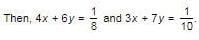Solving the two equations, we get.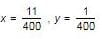∴ 1 woman's 1 day's work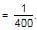⇒ 10 women's 1 day's work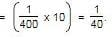Hence. 10 women will complete the work in 40 days

QUESTION: 12

A, B and C can complete a piece of work in 24, 6 and 12 days respectively. Working together, they will complete the same work in:

Solution:

A’s 1 day work = 1/24
B’s 1 day work = 1/6
C’s 1 day work = 1/12
(A+B+C)’s 1 day work = 1/24 + 1/6 + 1/12 = 7/24
The work will be completed by them is 24/7 days.

QUESTION: 13

A and B can complete a work in 15 days and 10 days respectively. They started doing the work together but after 2 days B had to leave and A alone completed the remaining work. The whole work was completed in :

Solution:

► Let total work be 30 units (LCM of 10 and 15).
► In one day, A can do 2 units of work and B can do 3 units of work.
► In one day, both A and B can do 5 units of work.
► In two days, A and B will complete 10 units of work. Remaining 20 units can be completed by A in 10 days (at rate of 2 units per day).
► Hence, whole work will be completed in 12 days.

QUESTION: 14

A machine P can print one lakh books in 8 hours, machine Q can print the same number of books in 10 hours while machine R can print them in 12 hours. All the machines are started at 9 A.M. while machine P is closed at 11 A.M. and the remaining two machines complete work. Approximately at what time will the work (to print one lakh books) be finished ?

Solution:

(P + Q + R)'s 1 hour's work = 1/8 + 1/10 + 1/12 = 37/120
Work done by P, Q and R in 2 hours = 37/120 x 2 = 37/60
Remaining work = 1 - 37/60 = 23/60
(Q + R)'s 1 hour's work = 1/10 + 1/12 = 11/60
Now, 11/60 work is done by Q and R in 1 hour.
So, 23/60 work will be done by Q and R in 60/11 x 23/60 = 23/11 hours ≌ 2 hours.
So, the work will be finished approximately 2 hours after 11 A.M., i.e., around 1 P.M.

QUESTION: 15

A can do a certain work in the same time in which B and C together can do it. If A and B together could do it in 10 days and C alone in 50 days, then B alone could do it in:

Solution:

► Let a, b and c be the fraction of work per day by A,B & C respectively.
c = 1/50
a + b = 1/10 = 5/50 .
a = b + c.
Therefore,
⇒ 2b + c = 5/50
⇒ 2b = 5/50 - 1/50 = 2/25
⇒ b = 1/25
B will do it in 25 days.

QUESTION: 16

A and B together can do a piece of work in 30 days. A having worked for 16 days, B finishes the remaining work alone in 44 days. In how many days shall B finish the whole work alone?

Solution:

Let A's 1 day's work = x and B's 1 day's work = y.
Then, x + y = 1/30 and 16x + 44y = 1
Solving these two equations, we get:
x = 1/60 and y = 1/60
∴ B's 1 day's work = 1/60
Hence, B alone shall finish the whole work in 60 days.

QUESTION: 17

A takes twice as much time as B or thrice as much time as C to finish a piece of work. Working together, they can finish the work in 2 days. B can do the work alone in:

Solution:

Suppose A, B and C take x, x/2 and x/3 days respectively to finish the work.
Then,1/x + 2/x + 3/x = 1/2
⇒ 6/x = 1/2
⇒ x = 12.
So, B takes (12/2) = 6 days to finish the work.

QUESTION: 18

P can complete a work in 12 days working 8 hours a day. Q can complete the same work in 8 days working 10 hours a day. If both P and Q work together, working 8 hours a day, in how many days can they complete the work?

Solution:

P can complete the work in (12 x 8) hrs. = 96 hrs.
Q can complete the work in (8 x 10) hrs. = 80 hrs.
∴ P's 1 hour's work = 1/96 and Q's 1 hour's work = 1/80
(P + Q)'s 1 hour's work = 1/96 + 1/80 = 11/480
So, both P and Q will finish the work in 480/11 hrs.
∴ Number of days of 8 hours each = 480/11 x 1/8 = 60/11 days

QUESTION: 19

A can finish a work in 24 days, B in 9 days and C in 12 days. B and C start the work but are forced to leave after 3 days. The remaining work was done by A in:

Solution:

(B + C)'s 1 day's work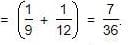Work done by B and C in 3 days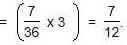Remaining work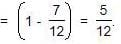Now, 1/24 work is done by A in 1 day.
So, 5/12 work is done by A in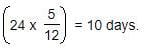QUESTION: 20

A is thrice as good as workman as B and therefore is able to finish a job in 60 days less than B. Working together, they can do it in:

Solution:

► Ratio of times taken by A and B = 1 : 3.
► The time difference is (3 - 1) 2 days while B take 3 days and A takes 1 day.
► If difference of time is 2 days, B takes 3 days.
► If difference of time is 60 days, B takes 3/2 x 60 = 90 days.
► So, A takes 30 days to do the work.
A's 1 day's work = 1/30
B's 1 day's work = 1/90
(A + B)'s 1 day's work = 1/30 + 1/90 = 4/90 = 2/45
∴ A and B together can do the work in 45/2 = 22.5 days.

QUESTION: 21

A does 80% of a work in 20 days. He then calls in B and they together finish the remaining work in 3 days. How long B alone would take to do the whole work?

Solution:

Whole work is done by A in 20 x 5/4 = 25 days.
Now,(1 - 4/5) i.e., 1/5 work is done by A and B in 3 days.
Whole work will be done by A and B in (3 x 5) = 15 days.
A's 1 day's work = 1/25, (A + B)'s 1 day's work = 1/15
∴ B's 1 day's work = 1/15 - 1/25 = 4/150 = 2/75
So, B alone would do the work in 75/2 = 37.5 days.

QUESTION: 22

If 6 men and 8 boys can do a piece of work in 10 days while 26 men and 48 boys can do the same in 2 days, the time taken by 15 men and 20 boys in doing the same type of work will be:

Solution:

► Let 1 man's 1 day's work = x and 1 boy's 1 day's work = y.
► Then, 6x + 8y = 1/10 and 26x + 48y = 1/2
Solving these two equations, we get :
x = 1/100 and y = 1/200
(15 men + 20 boy)'s 1 day's work = 15/100 + 20/200 = 1/4
∴ 15 men and 20 boys can do the work in 4 days.

QUESTION: 23

A and B can do a work in 8 days, B and C can do the same work in 12 days. A, B and C together can finish it in 6 days. A and C together will do it in :

Solution:

(A + B + C)'s 1 day's work = 1/6
(A + B)'s 1 day's work = 1/8
(B + C)'s 1 day's work = 1/12
Therefore (A + C)'s 1 day's work
⇒ (2 x 1/6) - (1/8 + 1/12)
⇒ (1/3 - 5/24)
⇒ 3/24
⇒ 1/8
So, A and C together will do the work in 8 days.

QUESTION: 24

A, B and C can do a piece of work in 20, 30 and 60 days respectively. In how many days can A do the work if he is assisted by B and C on every third day?

Solution:

A's 2 day's work = 1/20 x 2 = 1/10
(A + B + C)'s 1 day's work = (1/20 + 1/30 + 1/60) = 6/60 = 1/10
Work done in 3 days = 1/10 + 1/10 = 1/5
Now, 1/5 work is done in 3 days.
∴ Whole work will be done in (3 x 5) = 15 days.

QUESTION: 25

10 women can complete a work in 7 days and 10 children take 14 days to complete the work. How many days will 5 women and 10 children take to complete the work?

Solution:

1 woman's 1 day's work = 1/70
1 child's 1 day's work = 1/140
(5 women + 10 children)'s day's work = 5/170 + 10/140 = 1/14 + 1/14 = 1/7
∴ 5 women and 10 children will complete the work in 7 days.

QUESTION: 26

A is 30% more efficient than B. How much time will they, working together, take to complete a job which A alone could have done in 23 days?

Solution:

► Ratio of times taken by A and B = 100 :130 = 10:13
► Suppose B takes x days to do the work
Then,10:13::23:x
⇒ x = (23×13)/10
⇒ x = 299/10
A's 1day's work = 1/23
B's 1day's work = 10/299
(A + B)'s 1 day's work = 1/23 + 10/299 = 23/299 = 1/13
∴ A and B together can complete the work in 13 days.

QUESTION: 27

A and B can together finish a work 30 days. They worked together for 20 days and then B left. After another 20 days, A finished the remaining work. In how many days A alone can finish the work?

Solution:

(A + B)'s 20 day's work = 1/30 x 20 = 2/3
Remaining work =1 - 2/3 = 1/3
Now, 1/3 work is done by A in 20 days.
Therefore, the whole work will be done by A in (20 x 3) = 60 days.

QUESTION: 28

Twenty women can do a work in sixteen days. Sixteen men can complete the same work in fifteen days. What is the ratio between the capacity of a man and a woman?

Solution:

(20 x 16) women can complete the work in 1 day.
∴ 1 woman's 1 day's work =1/320
(16 x 15) men can complete the work in 1 day.
∴ 1 man's 1 day's work = 1/240
So, required ratio = 1/240 : 1/320
⇒ 1/3 :1/4
⇒ 4 : 3 (cross multiplied)

QUESTION: 29

Sakshi can do a piece of work in 20 days. Tanya is 25% more efficient than Sakshi. The number of days taken by Tanya to do the same piece of work is:

Solution:

Ratio of times taken by Sakshi and Tanya = 125 : 100 = 5 : 4.
Suppose Tanya takes x days to do the work.
5 : 4 :: 20 : x ⇒ x = 4 * (20/5)
⇒ x = 16 days
Hence, Tanya takes 16 days to complete the work.

QUESTION: 30

X can do a piece of work in 40 days. He works at it for 8 days and then Y finished it in 16 days. How long will they together take to complete the work?

Solution:

No of days taken by X to finish the work = 40
So part of work done by X in 1 day = 1/40
Part of work done by X in 8 days = 8/40 = 1/5
The remaining part = 1 - 1/5 = 4/5
No of days taken by Y to finish 4/5 part = 16
Part of work done by Y in 1 day = (4/5)/16
⇒ (4/5)(1/16)
⇒ 1/20
Part of work done by both together in 1 day = (1/40) + (1/20) = 3/40
Hence, X and Y will together complete the work in = 40/3Use Code STAYHOME200 and get INR 200 additional OFF Use Coupon Code